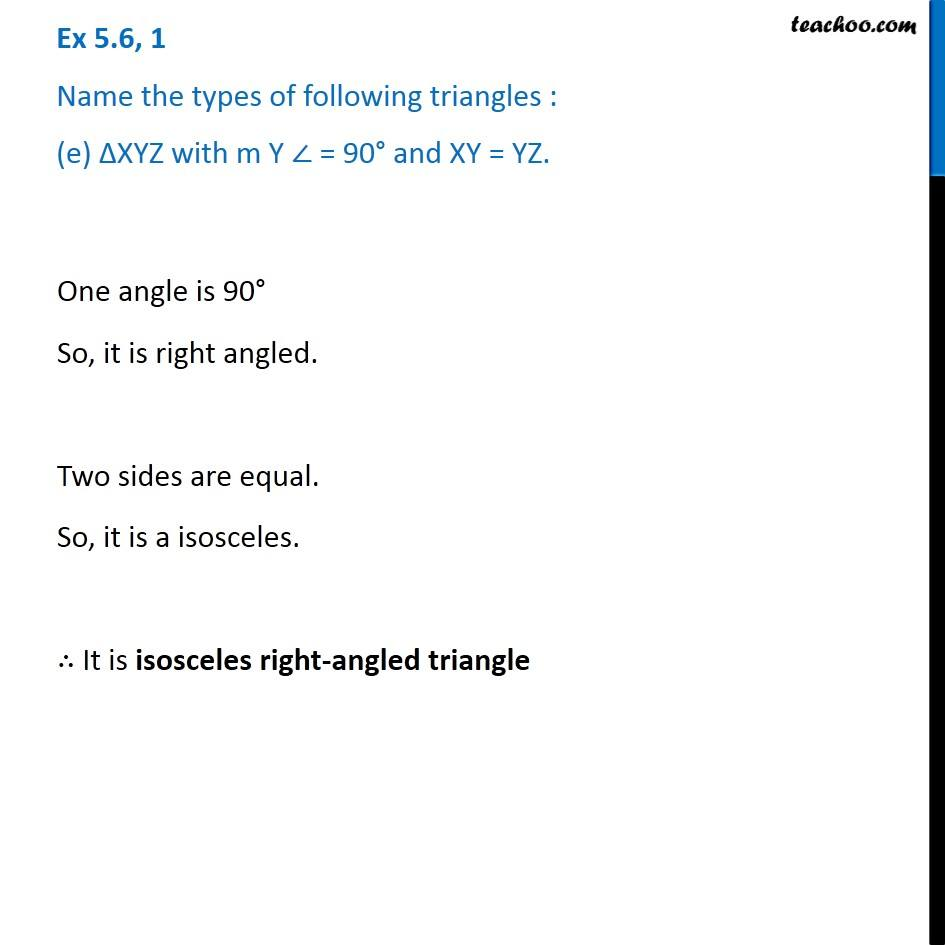1. Chapter 5 Class 6 Understanding Elementary Shapes
2. Serial order wise
3. Ex 5.6

Transcript

Ex 5.6, 1 Name the types of following triangles : (e) ∆XYZ with m Y ∠ = 90° and XY = YZ. One angle is 90° So, it is right angled. Two sides are equal. So, it is a isosceles. ∴ It is isosceles right-angled triangle